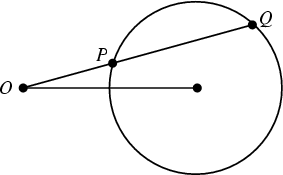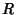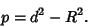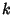## Power (Circle)The Power of the two pointsandwith respect to a Circle is defined byLetbe the Radius of a Circle andbe the distance between a pointand the circle's center. Then the Power of the pointrelative to the circle isIfis outside the Circle, its Power is Positive and equal to the square of the length of the segment fromto the tangent to the Circle through. Ifis inside the Circle, then the Power is Negative and equal to the product of the Diameters through.

The Locus of points having Powerwith regard to a fixed Circle of Radiusis a Concentric Circle of Radius. The Chordal Theorem states that the Locus of points having equal Power with respect to two given nonconcentric Circles is a line called the Radical Line (or Chordal; Dörrie 1965).

References

Coxeter, H. S. M. and Greitzer, S. L. Geometry Revisited. Washington, DC: Math. Assoc. Amer., pp. 27-31, 1967.

Dixon, R. Mathographics. New York: Dover, p. 68, 1991.

Dörrie, H. 100 Great Problems of Elementary Mathematics: Their History and Solutions. New York: Dover, p. 153, 1965.

Johnson, R. A. Modern Geometry: An Elementary Treatise on the Geometry of the Triangle and the Circle. Boston, MA: Houghton Mifflin, pp. 28-34, 1929.

Pedoe, D. Circles: A Mathematical View, rev. ed. Washington, DC: Math. Assoc. Amer., pp. xxii-xxiv, 1995.